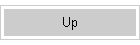VSWR Reactive PowerMany antennas and antenna designers neglect the true cause of loss. The major problem using short antennas is the reactance, not the length!

What is Reactance?

Reactance really means the voltage and current at that point of the system are no longer in-phase or 180 degrees out-of-phase. The result is, for the same real work power, the reactive power or Volt-Amperes-Reactive power is higher. We can say the "apparent power" is higher while the "power that does real work" remains the same.

Let's assume we have a transmitter with 100 watts output. With a zero reactance load the RMS voltage times RMS current will be 100. Remember the formula P= I E ? If the load is 50 ohms, we would have 70.7 volts times 1.414 amperes.

When we add reactance to the system, the voltage and current are no longer in step at that point in the system. To have 100 watts of real useful power, we might have 1000 volts and 2 amperes! That's 2kW VAR power with a 100W transmitter. This is really why feed lines get lossy and tuners burn up when an antenna system is very reactive.

What Does Reactive Power Do?

My first lesson in reactive power occurred around 1962 or 63. I was a Novice with a single 807 in my homebrew transmitter. It ran 60-75 watts input, or about 30-50 watts output. I tried to load my 40 meter dipole on 80 meters, and my feed line actually melted!

Let's look at an 80 meter dipole  example. With 100 watts and a modest height 80 meter dipole at resonance we have 1.32 amperes at 75.9 volts at the feedpoint. The 50 ohm SWR is 1.15:1

Frequency = 3.72 MHz
Source 1 Voltage = 75.89 V. at -0.1 deg.
Current = 1.318 A. at 0.0 deg.
Impedance = 57.59 - J 0.09832 ohms
Power = 100 watts
SWR (50 ohm system) = 1.152 (450 ohm system) = 7.813

Let's Shorten the Dipole....

For the 102-foot G5RV we have:

Frequency = 3.72 MHz
Source 1 Voltage = 143.5 V. at -61.09 deg.
Current = 1.441 A. at 0.0 deg.
Impedance = 48.16 - J 87.19 ohms
Power = 100 watts
SWR (50 ohm system) = 4.957 (450 ohm system) = 9.699

Notice if we multiply 1.441 amperes times 143.5 volts, we have 206.8 watts of reactive power.

With the dipole at G5RV length of 102 feet, we have 1.44 amperes and 143.5 volts. The VAR power is 206.6 watts with 100 watts of real power applied. The VSWR referenced to 50 ohms is 5:1. Losses are not significantly different, because VAR power is only 207 watts.

Let's Shorten the Dipole even more........

When we shorten the antenna to 88 feet, we have a problem.

Frequency = 3.72 MHz
Source 1 Voltage = 1078 V. at -87.53 deg.
Current = 2.153 A. at 0.0 deg.
Impedance = 21.58 - J 500.3 ohms
Power = 100 watts
SWR (50 ohm system) > 100 (450 ohm system) = 46.651

What a change in the system! With a 100 watt transmitter current is now 2.15 amperes while voltage is 1078 volts. 50 ohm SWR is over 100:1. Antenna feedpoint VAR power is 2317 watts with only 100 real watts applied. So far as the overall system, including the matching, feed line, conductors, and insulators are concerned, it is like we have increased power level 23 times.

Let's look at a 20 meter dipole on 80 meters.

Frequency = 3.72 MHz
Source 1 Voltage = 14570 V. at -89.94 deg.
Current = 6.233 A. at 0.0 deg.
Impedance = 2.574 - J 2338 ohms
Power = 100 watts
SWR (50 ohm system) > 100 (450 ohm system) > 100

VAR power is now 9.1 kilowatts with a 100 watt transmitter.

Conclusion

VAR power is the real killer in system efficiency and component damage. VAR power  is the real reason a very short dipole taxes system components and has terrible efficiency.

We can get away with a 88-foot dipole on 80 meters if we have proper reactance compensation. Proper reactance compensation brings voltage and current back into phase, reducing the apparent power handled by the system. This reduces losses.

The components required must be sized to handle the increased voltage and current. A 100 watt transmitter operating into a highly reactive load can be like running 9kW, so far as the components in the reactive part of the system are concerned. This means the feed line, balun, and tuner must be conservatively rated when using a short dipole, or you have to run very low power. It also means losses will be high. We have to use very low loss components when reactive power levels are high, otherwise we convert energy into heat.

If we look at the antenna by itself, we hardly notice any change in antenna performance when length is decreased from 130 feet to 50 feet or less on 80 meters. The problem is the reactive power of a very short antenna stresses other components, including the feed line, balun, and tuner.

We probably wouldn't notice 5dB of power loss, after all conditions can vary many decibel from day to day.  This is why QRP operators often enjoy using inefficient antennas. Operators who place their antennas in competitive situations would get the feeling something is not quite right.  Of course with QRO, poor efficiency shows up like a slap across the face. A melted tuner, balun, or feed line will pique someone's interest.

Power loss might easily slip under our "RADAR" at 100 watts or less, but at high power the additional component stresses will capture our attention!